# 4 5 Graphs of Sine and Cosine Functions

• Slides: 32
Download presentation4. 5 Graphs of Sine and Cosine Functions Copyright © Cengage Learning. All rights reserved.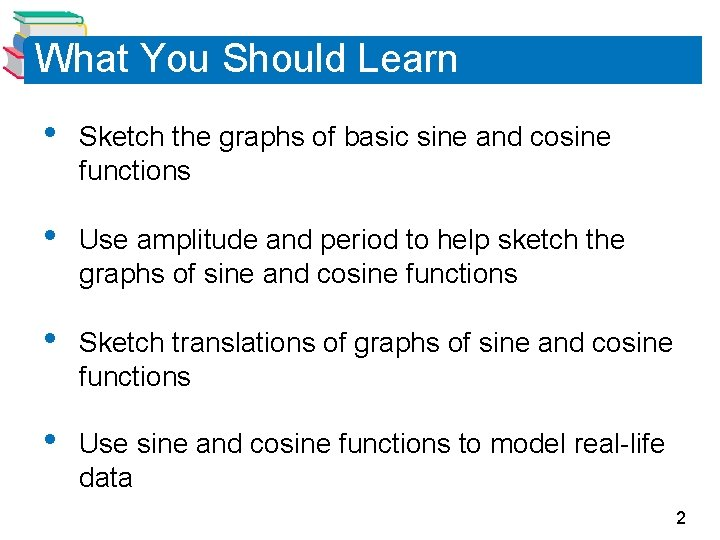What You Should Learn • Sketch the graphs of basic sine and cosine functions • Use amplitude and period to help sketch the graphs of sine and cosine functions • Sketch translations of graphs of sine and cosine functions • Use sine and cosine functions to model real-life data 2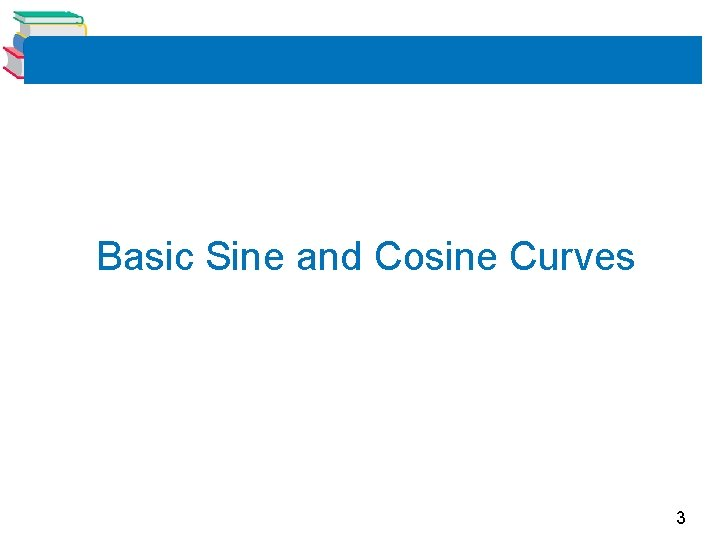Basic Sine and Cosine Curves 3Basic Sine and Cosine Curves The graph of the sine function is a sine curve. I drew this curve using the table on your unit circle. In Figure 4. 43, the black portion of the graph represents one period of the function and is called one cycle of the sine curve. Figure 4. 43 The gray portion of the graph indicates that the basic sine wave repeats indefinitely to the right and left. 4Basic Sine and Cosine Curves The graph of the cosine function is shown in Figure 4. 44. This was also drawn using points from the table on your unit circle handout. Figure 4. 44 5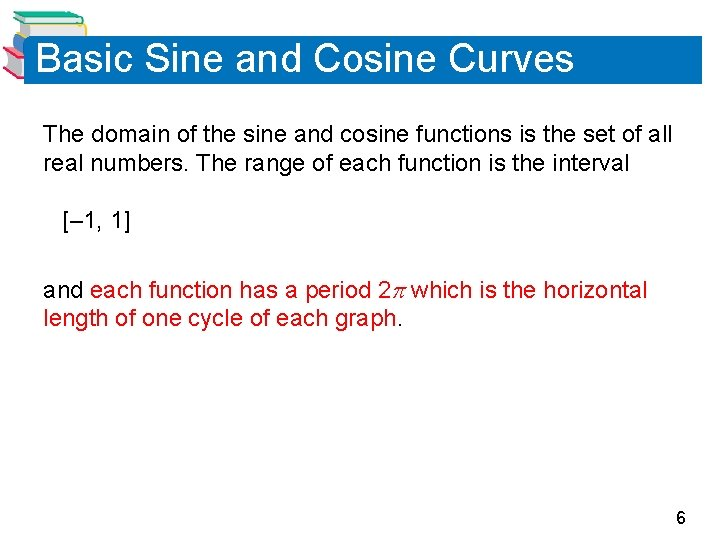Basic Sine and Cosine Curves The domain of the sine and cosine functions is the set of all real numbers. The range of each function is the interval [– 1, 1] and each function has a period 2 which is the horizontal length of one cycle of each graph. 6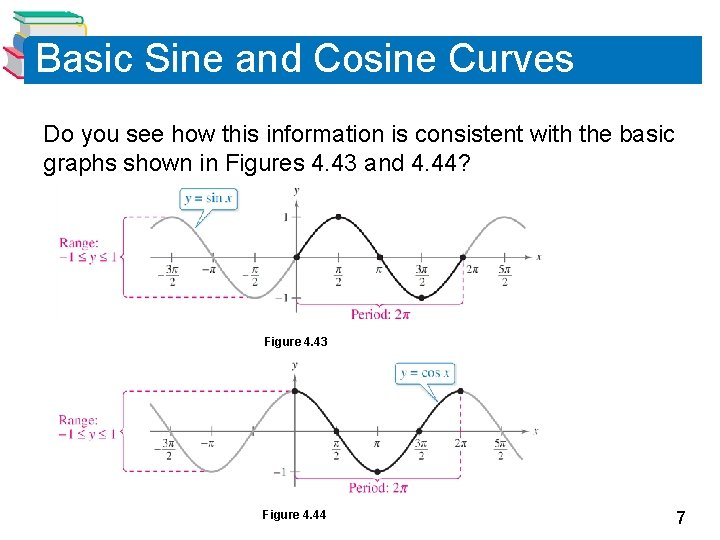Basic Sine and Cosine Curves Do you see how this information is consistent with the basic graphs shown in Figures 4. 43 and 4. 44? Figure 4. 43 Figure 4. 44 7Basic Sine and Cosine Curves To sketch the graphs of the basic sine and cosine functions by hand, it helps to note five key points in one period of each graph: the intercepts, the maximum points, and the minimum points. We will call this the Five Point Method. The table below lists the five key points on the graphs of y = sin x and y = cos x. 8Basic Sine and Cosine Curves Note in Figures 4. 43 and 4. 44 that the sine curve is symmetric with respect to the origin, whereas the cosine curve is symmetric with respect to the y-axis. These properties of symmetry follow from the fact that the sine function is odd whereas the cosine function is even. 9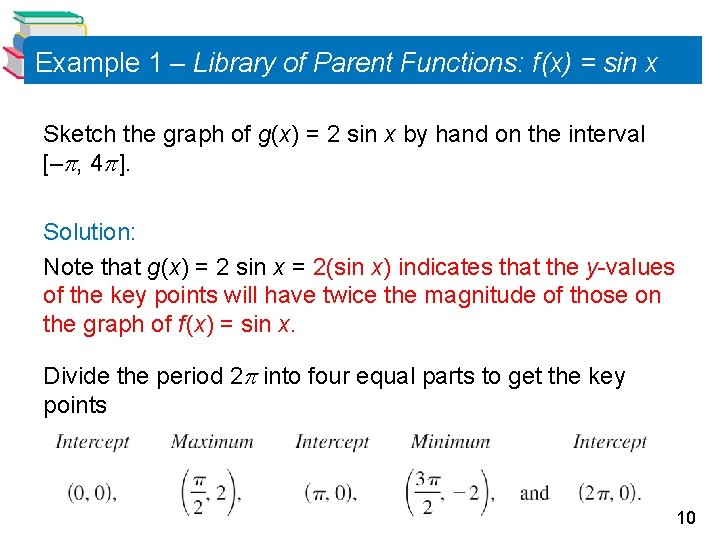Example 1 – Library of Parent Functions: f (x) = sin x Sketch the graph of g(x) = 2 sin x by hand on the interval [– , 4 ]. Solution: Note that g(x) = 2 sin x = 2(sin x) indicates that the y-values of the key points will have twice the magnitude of those on the graph of f (x) = sin x. Divide the period 2 into four equal parts to get the key points 10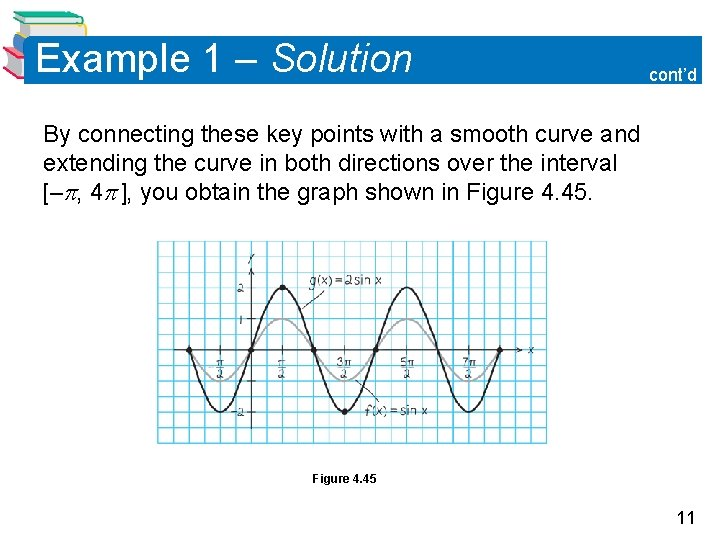Example 1 – Solution cont’d By connecting these key points with a smooth curve and extending the curve in both directions over the interval [– , 4 ], you obtain the graph shown in Figure 4. 45 11Amplitude and Period of Sine and Cosine Curves 12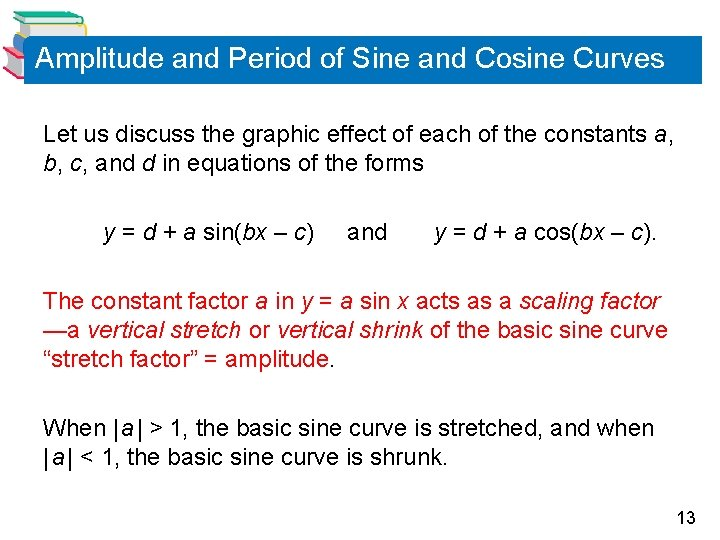Amplitude and Period of Sine and Cosine Curves Let us discuss the graphic effect of each of the constants a, b, c, and d in equations of the forms y = d + a sin(bx – c) and y = d + a cos(bx – c). The constant factor a in y = a sin x acts as a scaling factor —a vertical stretch or vertical shrink of the basic sine curve “stretch factor” = amplitude. When | a | > 1, the basic sine curve is stretched, and when | a | < 1, the basic sine curve is shrunk. 13Amplitude and Period of Sine and Cosine Curves The result is that the graph of y = a sin x ranges between –a and a instead of between – 1 and 1. The absolute value of a is the amplitude of the function y = a sin x. The range of the function y = a sin x for a > 0 is –a y a. 14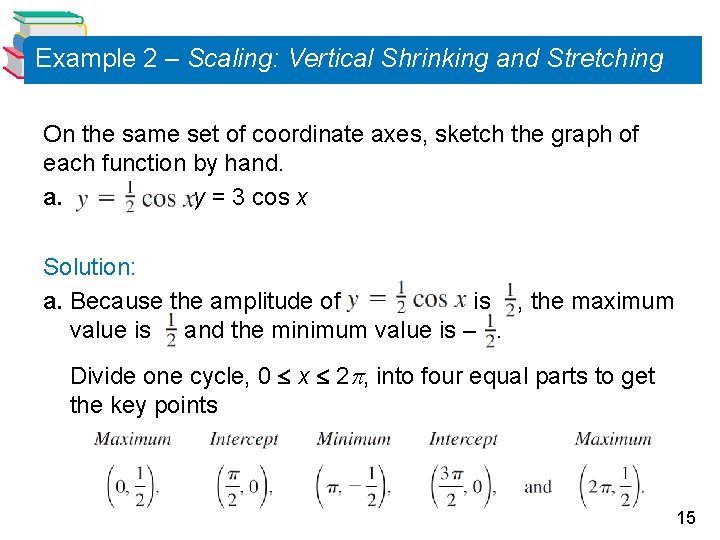Example 2 – Scaling: Vertical Shrinking and Stretching On the same set of coordinate axes, sketch the graph of each function by hand. a. b. y = 3 cos x Solution: a. Because the amplitude of is , the maximum value is and the minimum value is –. Divide one cycle, 0 x 2 , into four equal parts to get the key points 15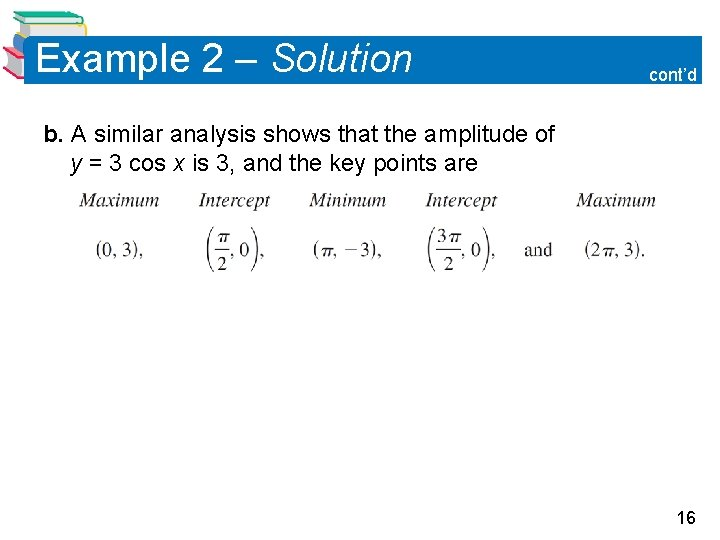Example 2 – Solution cont’d b. A similar analysis shows that the amplitude of y = 3 cos x is 3, and the key points are 16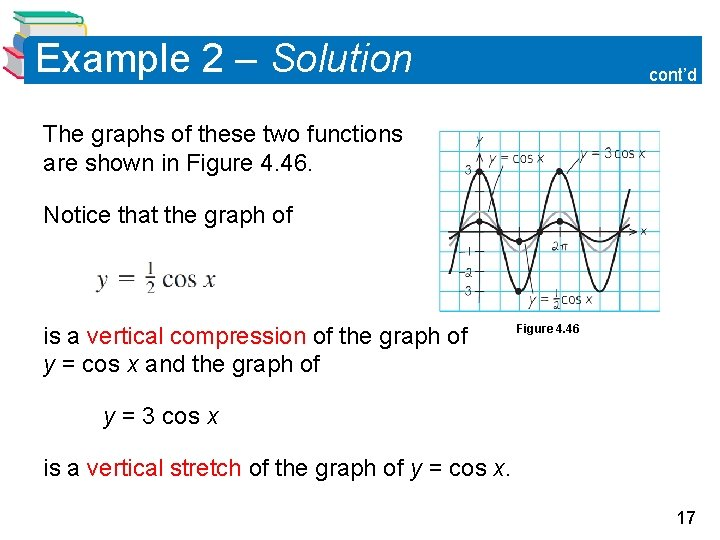Example 2 – Solution cont’d The graphs of these two functions are shown in Figure 4. 46. Notice that the graph of is a vertical compression of the graph of y = cos x and the graph of Figure 4. 46 y = 3 cos x is a vertical stretch of the graph of y = cos x. 17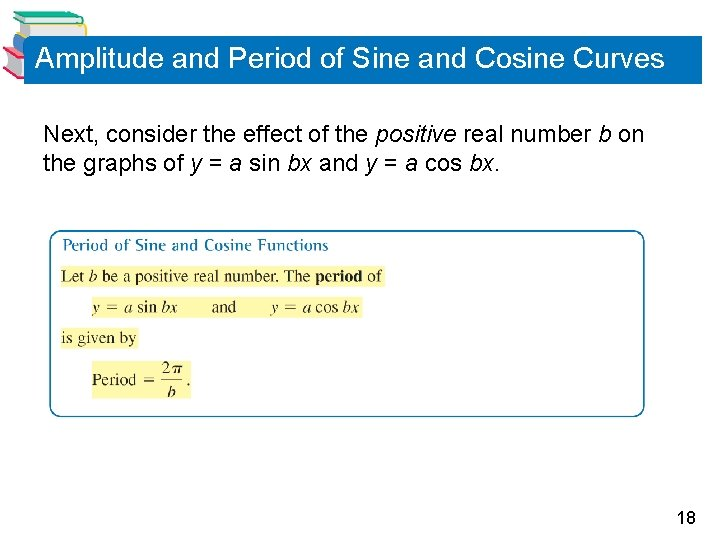Amplitude and Period of Sine and Cosine Curves Next, consider the effect of the positive real number b on the graphs of y = a sin bx and y = a cos bx. 18Amplitude and Period of Sine and Cosine Curves Note that when 0 < b < 1, the period of y = a sin bx is greater than 2 and represents a horizontal stretching of the graph of y = a sin x. Similarly, when b > 1, the period of y = a sin bx is less than 2 and represents a horizontal shrinking of the graph of y = a sin x. 19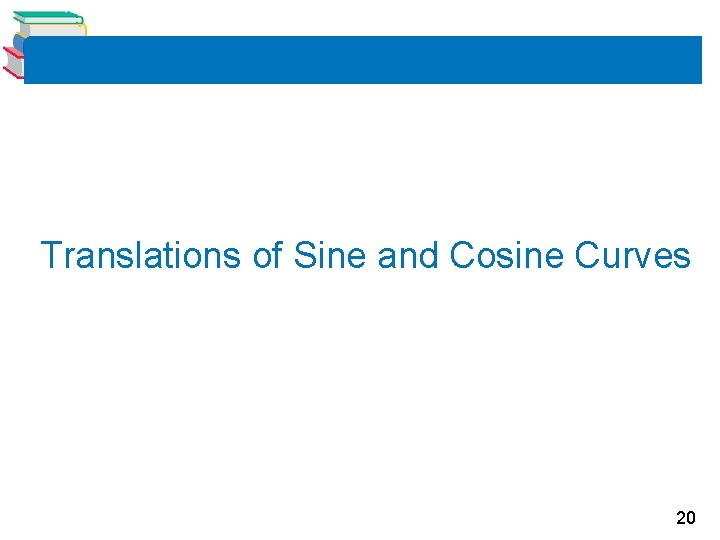Translations of Sine and Cosine Curves 20Translations of Sine and Cosine Curves The constant c in the general equations y = a sin(bx – c) and y = a cos(bx – c) creates horizontal translations (shifts) of the basic sine and cosine curves. To find the horizontal shift, first factor b out of the parentheses. 21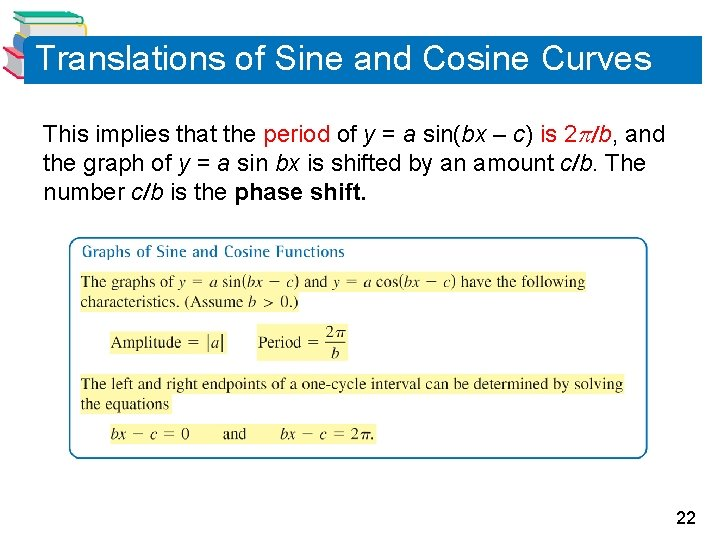Translations of Sine and Cosine Curves This implies that the period of y = a sin(bx – c) is 2 b, and the graph of y = a sin bx is shifted by an amount c b. The number c b is the phase shift. 22Example 4 – Horizontal Translation Analyze the graph of Solution: The amplitude is the period is 2. , the horizontal shift is to the right, and Therefore, the interval which corresponds to one cycle of the graph is 23Example 4 – Solution cont’d Dividing this interval into four equal parts produces the following key points. 24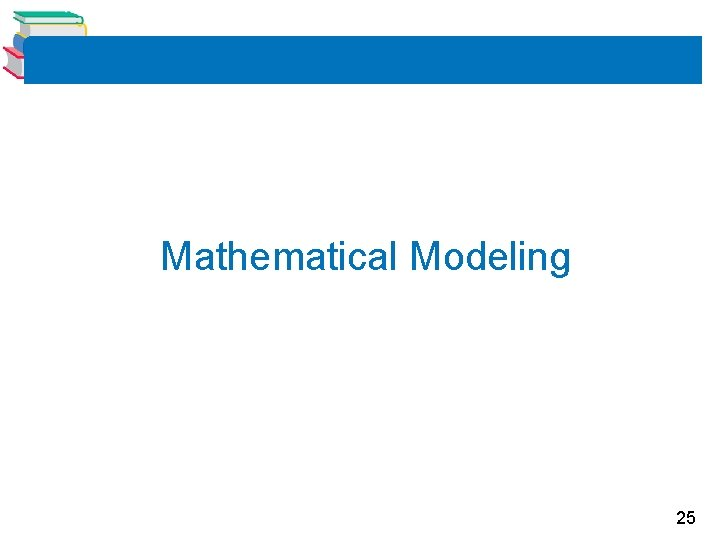Mathematical Modeling 25Mathematical Modeling Read this slide and those that follow, but do not copy them down. Sine and cosine functions can be used to model many real-life situations, including electric currents, musical tones, radio waves, tides, and weather patterns. 26Example 8 – Finding a Trigonometric Model Throughout the day, the depth of the water at the end of a dock varies with the tides. The table shows the depths (in feet) at various times during the morning. 27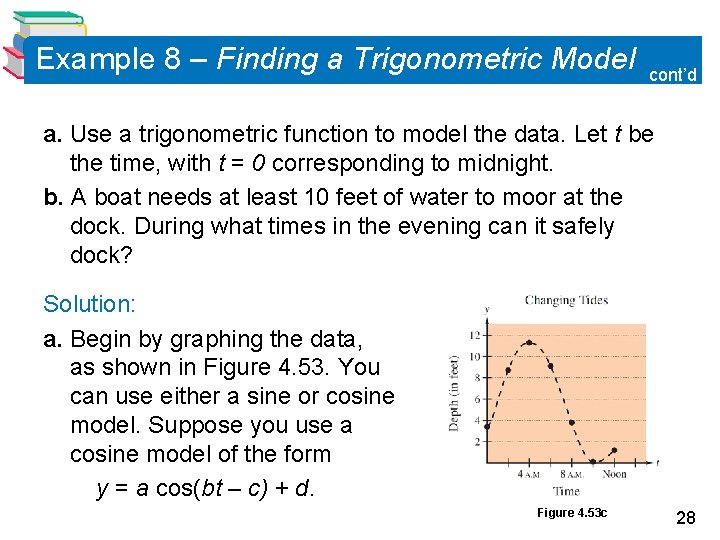Example 8 – Finding a Trigonometric Model cont’d a. Use a trigonometric function to model the data. Let t be the time, with t = 0 corresponding to midnight. b. A boat needs at least 10 feet of water to moor at the dock. During what times in the evening can it safely dock? Solution: a. Begin by graphing the data, as shown in Figure 4. 53. You can use either a sine or cosine model. Suppose you use a cosine model of the form y = a cos(bt – c) + d. Figure 4. 53 c 28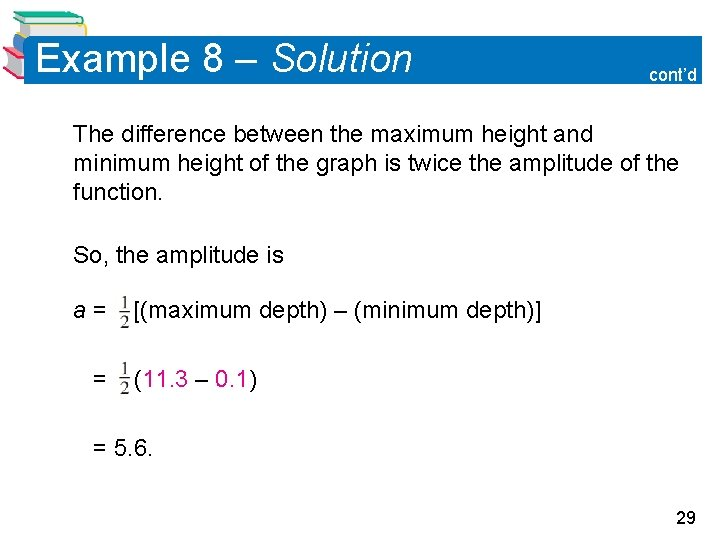Example 8 – Solution cont’d The difference between the maximum height and minimum height of the graph is twice the amplitude of the function. So, the amplitude is a= = [(maximum depth) – (minimum depth)] (11. 3 – 0. 1) = 5. 6. 29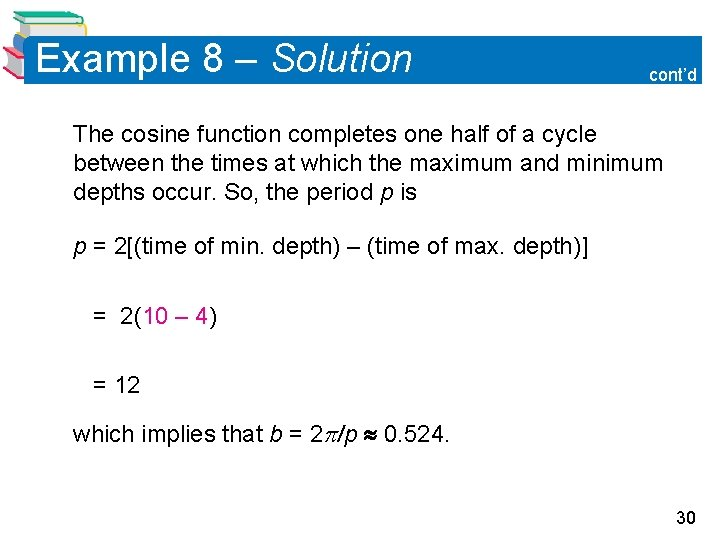Example 8 – Solution cont’d The cosine function completes one half of a cycle between the times at which the maximum and minimum depths occur. So, the period p is p = 2[(time of min. depth) – (time of max. depth)] = 2(10 – 4) = 12 which implies that b = 2 p 0. 524. 30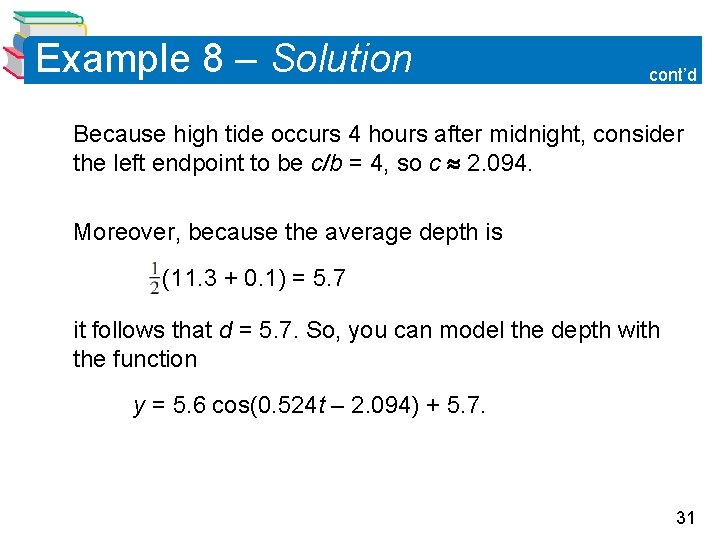Example 8 – Solution cont’d Because high tide occurs 4 hours after midnight, consider the left endpoint to be c b = 4, so c 2. 094. Moreover, because the average depth is (11. 3 + 0. 1) = 5. 7 it follows that d = 5. 7. So, you can model the depth with the function y = 5. 6 cos(0. 524 t – 2. 094) + 5. 7. 31Example 8 – Solution cont’d b. Using a graphing utility, graph the model with the line y = 10. Using the intersect feature, you can determine that the depth is at least 10 feet between 2: 42 P. M. (t 14. 7) and 5: 18 P. M. (t 17. 3), as shown in Figure 4. 54 32